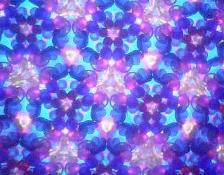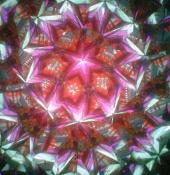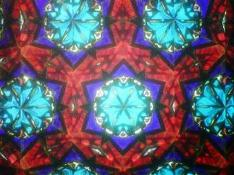## Kaleidoscope Mirror Systems - 3-Mirror Systems

3-Mirror Systems

The 3-mirror system is configured in a triangular shape.  Alll three sides are mirrors.  With this system, images are reflected throughout the entire field of view producing honeycomb-like patterns.

There are four comon configurations of 3-mirror systems in order of popularity:

• The 60-60-60 Equilateral Triangle
• The 30-60-90 Right triangle
• The Isosceles Triangle (has two equal sides)
• The 45-45-90 Isosceles Right Triangle

Below are the characteristics of each: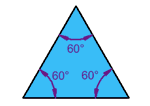### Equilateral Triangle

Three equal sides
Three equal angles, always 60°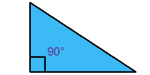### Right Triangle

No equal sides
No equal angles, one 90°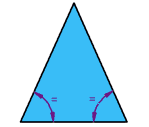### Isosceles Triangle

Two equal sides
Two equal angles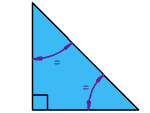### Isosceles Right Triangle

Two equal sides
Two equal angles,  one 90°

Each of these images would be repeated throughout the entire field of view.

Below are examples of the three most popular types of 3-mirror systems (the 60-60-60 equilateral triangle, the isosceles triangle and the 30-60-90 triangle) .  Notice that the last scope is a view of the mirrors after the wheels were removed (the second photo shows the 30-60-90 scope's mirror system up close).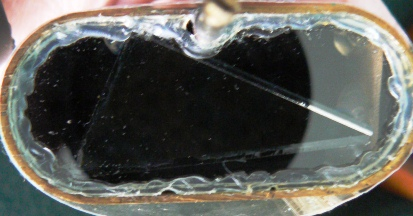Below are the images from the 3 scopes (order - equilateral triangle, isosceles triangle and the 30-60-90 triangle).